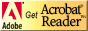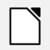# PC474 Optical Networks Laboratory

## Power Measurements

### Objectives

1. To review the measurement of power in optical systems.

### Background

Power measurements are important in any situation involving data transmission.

Power is often expressed in decibels, where
PdB = 10 log10P

Logarithms make the units involved ambiguous, so in order to relate this to actual units, power can be expressed in dBm, where the power is measured in milliWatts. Thus
PdBm = 10 log10P(mW)

To convert back from dBm to mW, it should be obvious that
Pout (mW) = 10PdBm/10

Certain devices, such as amplifiers, can produce gain in a signal, meaning the output is greater than the input. In these cases, the gain is given by
A = Pout/Pin

It's often convenient to express gain in decibels, so
GdB = 10 log10(Pout/Pin)

In that case, to get the gain from the gain in decibels,
Pout/Pin = 10PdB/10
(Note that in this case Pout should be greater than Pin.)

Many things cause loss in a signal, and loss can be considered as negative gain, so
LdB = - 10 log10(Pout/Pin)

In that case, to get the loss from the loss in decibels,
Pout/Pin = 10- PdB/10
(Note that in this case Pout must be less than Pin.)

Attenuation loss is dependent on wavelength. For radio frequencies,
Lattenuation-dB = 10 log10( (4 Π d)/λ2)
where d is the distance and λ is the wavelength.

#### Why use decibels?

At first glance, using decibels seems more complicated than using "normal" power units, such as Watts. However, when there are several elements in a system all producing gain or loss in a signal, it gets more complex. To get the final output of a system with several gains and losses, the original signal must be multiplied by the gain (or loss) of each element in the system. This can be tedious.
On the other hand, if you express everything in dB (or dBm), then gains and losses are represented by additions and subtractions.

### Procedure

1. Fill in the missing quantities in the following table:
Power in (mW) Power out (mW) Gain (or loss) (dB) Table 1: Power Measurements 100 10 15 0.2 50 10 0.01 -30 Related to those above 10 100 2 150 0.01 30

2. In many situations, rather than having actual power measurements, it's easier to use percent (or fractional) changes in power. Fill in the missing quantities in the following table:
Percent change (%) Fractional change Gain (or loss) (dB) Table 2: Power Measurements 90 0.9 10 0.1 2 0.01 5 1/2 0.001

Before you leave the lab, have the lab instructor sign your lab notebook immediately after your last entry.

## ResourcesIf you need to update a browser, you might try Firefox which is
• free
• open source
• available for several platformsSince this page uses cascading style sheets for its layout, it will look best with a browser which supports the specifications as fully as possible.

If you are looking for an office package, with a word processor, spreadsheet, etc., you might try LibreOffice which is
• free
• open source
• available for several platformsGo to the main page for the Department of Physics and Computer Science.

Wilfrid Laurier University